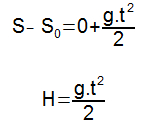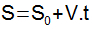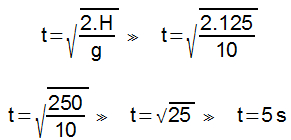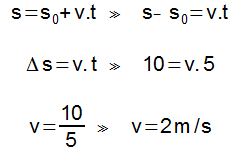Mechanics

# Horizontal launch

In the horizontal launch, an object launched horizontally executes a curvilinear movement, which is the result of two movements: one horizontally and one vertically.

Imagine the motion of a ball rolling across a table until it lands on the floor. When this ball leaves the table, it follows a curvilinear trajectory until it touches the ground. This type of movement is called horizontal launch and occurs when the object, initially with a horizontal velocity, is launched at a certain height.

we see that, when leaving the table, the ball starts a free fall movement because of the action of the force of gravity . Thus, we can conclude that an object launched horizontally has two speeds: one oriented horizontally and the other vertically, as shown in the following figure.

The horizontal velocity, V X , is constant throughout the movement, so we can say that, horizontally, the object performs a uniform rectilinear movement . Vertically, as it falls, the object’s velocity becomes greater and greater because of the acceleration of gravity , so on the y-axis, the motion is rectilinear and uniformly varied .

If, at the exact moment when the ball leaves the table with a certain horizontal velocity, another object is dropped vertically, so that it performs a free fall movement, the two bodies will touch the ground at the same time, as we can see in the image Next:

This happens because the two objects are launched at the same height and perform the same vertical movement, therefore, they will have the same fall time.

Equations

Vertical movement

Observing vertical motion, the position time function can be applied to uniformly varied rectilinear motion , as shown below:

Knowing that the initial velocity in the horizontal is zero, that the acceleration under which the object is subjected is that of gravity and that the difference between the final position and the initial position corresponds to the height (H) of the fall (S – S 0 = H), we can write:Horizontal movement

With horizontal movement, the position time function can be applied to uniform rectilinear movement and determine the range of an object thrown horizontally, according to the following formula:Knowing that the difference between the final position and the initial position, on the x axis, corresponds to the horizontal reach itself (A), we have that:Example

(Unic-MT) Consider a stone being thrown horizontally from the top of a 125.0 m high building, at a location where the magnitude of the acceleration of gravity is equal to 10 m/s 2 and having a horizontal reach equal to 10 .0 m. Under these conditions, it is concluded that the speed with which the stone was thrown, in m/s, is equal to

01. 2

02. 3

03. 4

04.5

05. 6

First, the stone’s fall time must be determined. This time will be found through the equation that determines the fall time in the free fall motion.With the time taken by the object to reach the ground, the launch velocity can be determined through the position time function for uniform rectilinear motion.